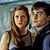# Can You Outsmart AI on This Difficult Math Quiz?

## AI is smart, but are you smarter?! Let's find out through this quiz complete with AI generated math questions. Let's go!Created by Carly Wallace
On Oct 23, 2023

Ai is a pretty complex system but with complexity comes faults. AI isn't perfect and even though it can generate basically anything, it doesn't mean it's always right. We've generated some AI math questions to see if you can beat it with your human intelligence. Let's find out how smart you really are.

>> Only GenZ Can Pass This 'Secrets of AI Quiz', Can You?

1 / 10

What is 12 x 9?

2 / 10

What is the greatest common divisor (GCD) of 18 and 24?

3 / 10

If you have \$200 and spend \$50, how much money do you have left?

4 / 10

If you roll a fair six-sided die, what is the probability of rolling a 3?

5 / 10

What is the area of a rectangle with a width of 5 and length of 8?

6 / 10

Calculate the mean (average) of the numbers 4, 7, 12, 5, and 9:

7 / 10

Solve the quadratic equation 2x^2 - 9x + 7 = 0

8 / 10

What is 15 divided by 3?

9 / 10

Solve for x: 3x - 7 = 14

10 / 10

If you flip a fair coin twice, what is the probability of getting heads both times?

10
Questions left

### How often do you use AI?

Calculating results
These are 10 of the World CRAZIEST Ice Cream Flavors
Created by Tal Garner
On Nov 18, 2021